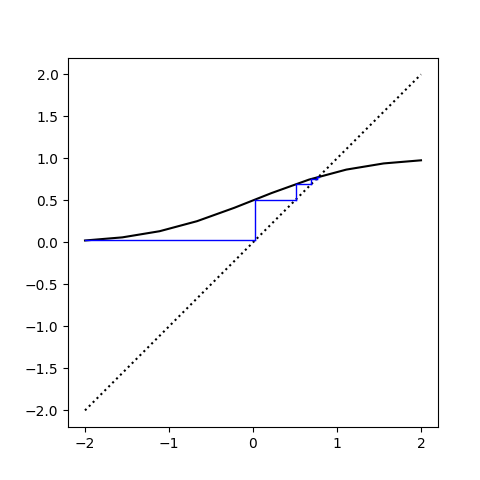# Normal probability fixed point

Let Z be a standard normal random variable. Then there is a unique x such that

Pr(Z < x) = x.

That is, Φ has a unique fixed point where Φ is the CDF of a standard normal.

It’s easy to find the fixed point: start anywhere and iterate Φ.

Here’s a cobweb plot that shows how the iterates converge, starting with -2.The black curve is a plot of Φ. The blue stairstep is a visualization of the iterates. The stairstep pattern comes from outputs turning into inputs. That is, vertical blue lines connect and input value x to its output value y, and the horizontal blue lines represent sliding a y value over to the dotted line y = x in order to turn it into the next x value.

y‘s become x‘s. The blue lines get short when we’re approaching the fixed point because now the outputs approximately equal the inputs.

Here’s a list of the first 10 iterates.

```    0.02275
0.50907
0.69465
0.75636
0.77528
0.78091
0.78257
0.78306
0.78320
0.78324
```

So it seems that after 10 iterations, we’ve converged to the fixed point in the first four decimal places.

## One thought on “Normal probability fixed point”

1. Serge Terekhov